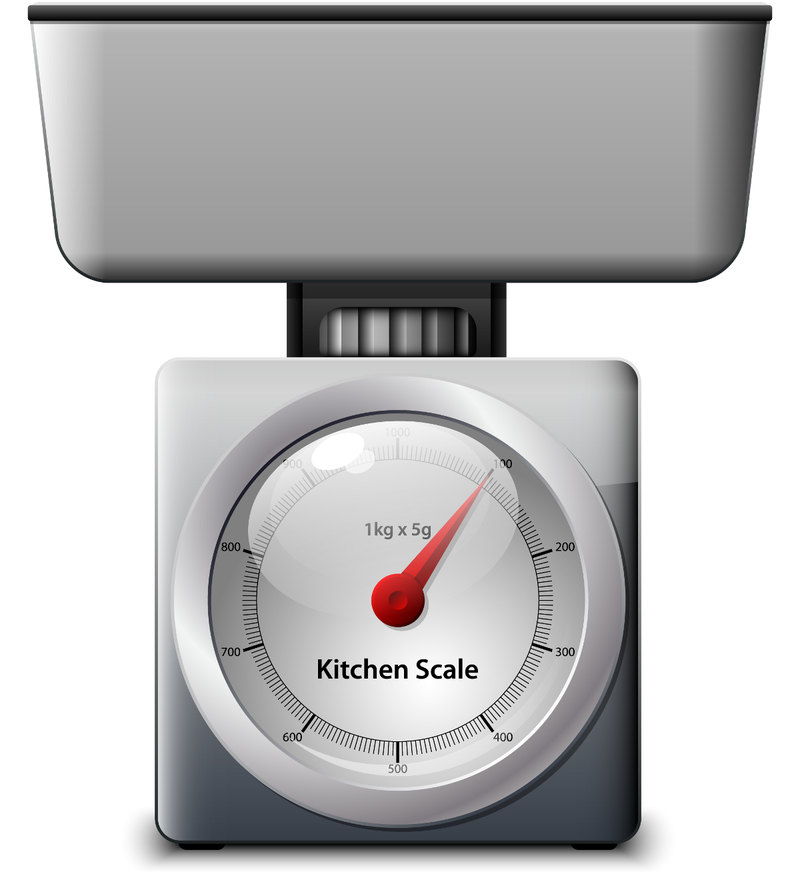## Introduction to Matter (Book)### Properties of Matter

#### - Mass versus Weight

The more matter an object contains, generally the more it weighs. However, weight is not the same thing as mass. Weight is a measure of the force of gravity pulling on an object. It is measured with a scale, like the kitchen scale in Figure below. The scale detects how forcefully objects in the pan are being pulled downward by the force of gravity. The SI unit for weight is the newton (N). The common English unit is the pound (lb). With Earth’s gravity, a mass of 1 kg has a weight of 9.8 N (2.2 lb).This kitchen scale measures weight. How does weight differ from mass?